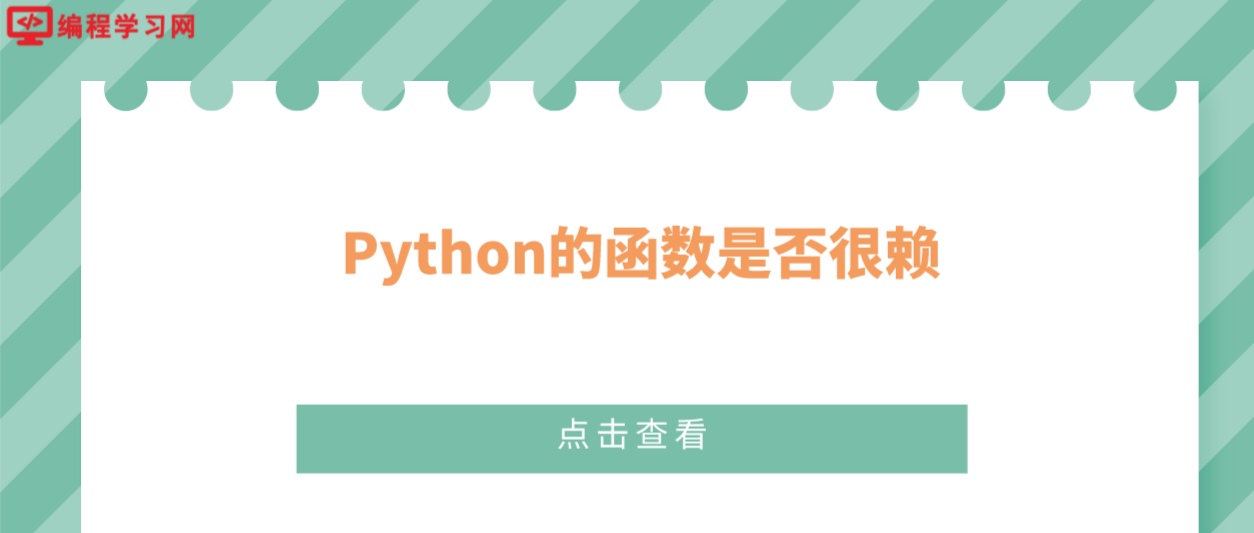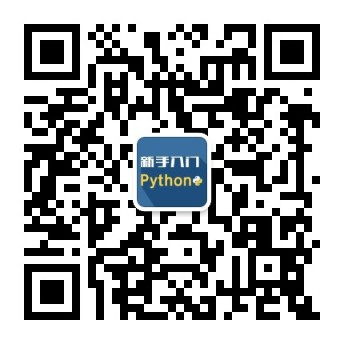2022
08-16

# Python的函数是否很赖### 第 1 题：万物皆对象

```print(isinstance(object, type))
print(isinstance(type, object))
print(isinstance(type, type))
print(isinstance(object, object))```

`>>> print(isinstance(object, type)) True >>> print(isinstance(type, object)) True >>> print(isinstance(type, type)) True >>> print(isinstance(object, object)) True >>>`

### 第 2 题：sum 的结果

```print(sum(""))
print(sum("", []))
print(sum("", {}))```

```>>> print(sum("")) 0 >>> print(sum("", []))
[] >>> print(sum("", {}))
{}
>>>```

`sum(iterable,/, start = 0)`

sum 从左到右开始对可迭代的项目进行求和，并返回求和的结果。但是当 iterable 为空时，返回 start 的值。由于 "" 是空字符串，因此总是返回 start 的值，start 的值如果不传入，那就会返回 start 的默认值 0.

### 第 3 题：Python 的函数懒吗？

```class follow:    def func(self):       return fol()
a = follow()
fol= int
print(a.func())```

```>>> class follow: ...    def func(self): ...       return fol()
... >>> a = follow() >>> fol = int >>> a.func() 0 >>>```

### 第 4 题：会不会报错

`print(sum([a.imag for a in [0,5,10e9,float('inf'),float('nan')]]))`

### 第 5 题：这符合数学常识吗：

`a=(1 << 53)+1 print(a+1.0 > a)`

`>>> 1 << 53 9007199254740992 >>> _ + 1 9007199254740993 >>> _ + 1.0 9007199254740992.0 >>>`